Next: The Schrödinger Equation Up: The Motivation for Quantum Previous: Quantization of Electronic Angular

## Wave-Particle Duality

Einstein had shown that the momentum of a photon is
(4)

This can be easily shown as follows. Assuming
for a photon and
for an electromagnetic wave, we obtain
(5)

Now we use Einstein's relativity result E = m c2 to find
(6)

which is equivalent to equation (4). Note that mrefers to the relativistic mass, not the rest mass, since the rest mass of a photon is zero. Since light can behave both as a wave (it can be diffracted, and it has a wavelength), and as a particle (it contains packets of energy
), de Broglie reasoned in 1924 that matter also can exhibit this wave-particle duality. He further reasoned that matter would obey the same equation (4) as light. In 1927, Davisson and Germer observed diffraction patterns by bombarding metals with electrons, confirming de Broglie's proposition.

de Broglie's equation offers a justification for Bohr's assumption (2). If we think of an electron as a wave, then for the electron orbit to be stable the wave must complete an integral number of wavelengths during its orbit. Otherwise, it would interfere destructively with itself. This condition may be written as
(7)

If we use the de Broglie relation (4), this can be rewritten as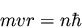(8)

which is identical to Bohr's equation (2).

Although de Broglie's equation justifies Bohr's quantization assupmtion, it also demonstrates a deficiency of Bohr's model. Heisenberg showed that the wave-particle duality leads to the famous uncertainty principle
(9)

One result of the uncertainty principle is that if the orbital radius of an electron in an atom r is known exactly, then the angular momentum must be completely unknown. The problem with Bohr's model is that it specifies r exactly and it also specifies that the orbital angular momentum must be an integral multiple of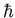. Thus the stage was set for a new quantum theory which was consistent with the uncertainty principle.Next: The Schrödinger Equation Up: The Motivation for Quantum Previous: Quantization of Electronic Angular